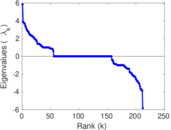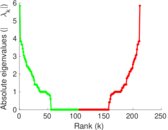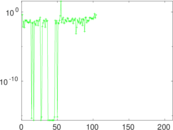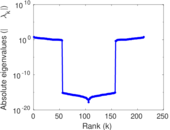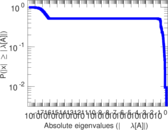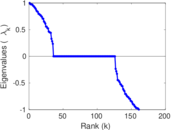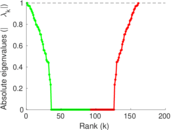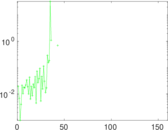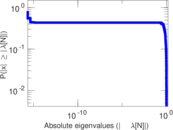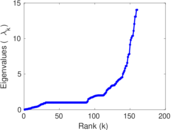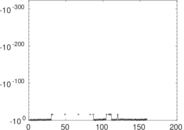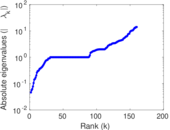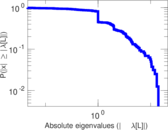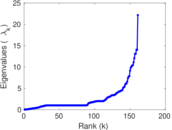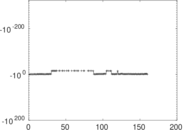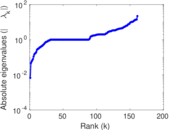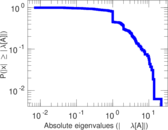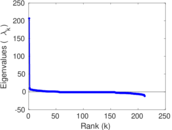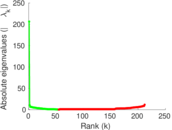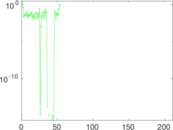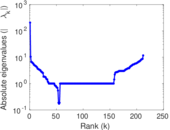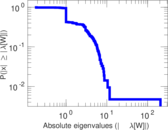# PDZBase

This is a network of protein–protein interactions from PDZBase.

 Code `MP` Internal name `maayan-pdzbase` Name PDZBase Data source http://research.mssm.edu/maayan/datasets/qualitative_networks.shtml AvailabilityDataset is available for download Consistency checkDataset passed all tests Category Metabolic network Node meaning Protein Edge meaning Interaction Network format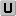Unipartite, undirected Edge typeUnweighted, no multiple edges Loops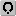Contains loops

## Statistics

 Size n = 212 Volume m = 244 Loop count l = 2 Wedge count s = 1,049 Claw count z = 3,221 Cross count x = 9,571 Triangle count t = 1 Square count q = 122 4-Tour count T4 = 5,656 Maximum degree dmax = 21 Average degree d = 2.301 89 Fill p = 0.010 807 0 Size of LCC N = 161 Diameter δ = 10 50-Percentile effective diameter δ0.5 = 4.718 88 90-Percentile effective diameter δ0.9 = 7.206 09 Median distance δM = 5 Mean distance δm = 5.114 56 Gini coefficient G = 0.442 178 Balanced inequality ratio P = 0.317 623 Relative edge distribution entropy Her = 0.924 723 Power law exponent γ = 3.006 86 Tail power law exponent γt = 2.091 00 Tail power law exponent with p γ3 = 2.091 00 p-value p = 0.009 000 00 Degree assortativity ρ = −0.356 716 Degree assortativity p-value pρ = 5.712 88 × 10−16 Clustering coefficient c = 0.002 859 87 Spectral norm α = 5.840 66 Algebraic connectivity a = 0.045 973 0 Spectral separation |λ1[A] / λ2[A]| = 1.000 15 Non-bipartivity bA = 0.000 152 507 Normalized non-bipartivity bN = 0.002 569 68 Algebraic non-bipartivity χ = 0.006 661 35 Spectral bipartite frustration bK = 0.000 641 433 Controllability C = 100 Relative controllability Cr = 0.471 698

## Plots

### Fruchterman–Reingold graph drawing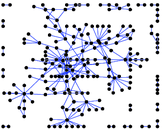### Degree distribution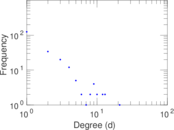### Cumulative degree distribution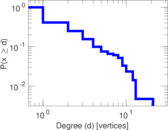### Lorenz curve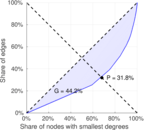### Spectral distribution of the adjacency matrix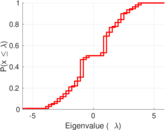### Spectral distribution of the normalized adjacency matrix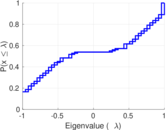### Spectral distribution of the Laplacian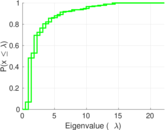### Spectral graph drawing based on the adjacency matrix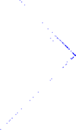### Spectral graph drawing based on the Laplacian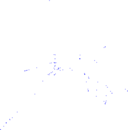### Spectral graph drawing based on the normalized adjacency matrix### Degree assortativity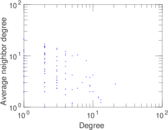### Zipf plot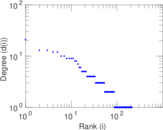### Hop distribution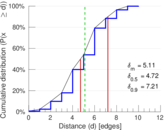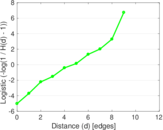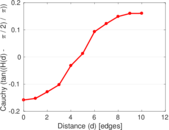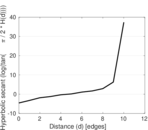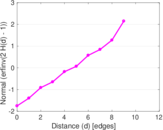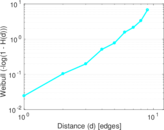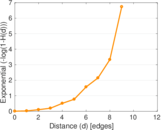### Double Laplacian graph drawing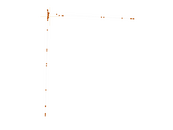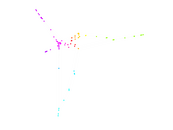### Delaunay graph drawing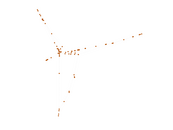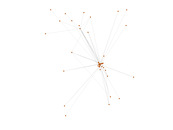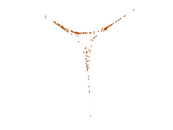### Clustering coefficient distribution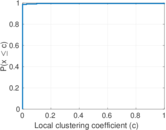### Average neighbor degree distribution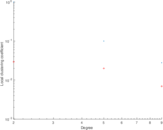### SynGraphy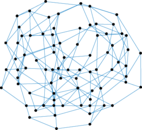### Matrix decompositions plots